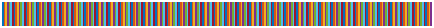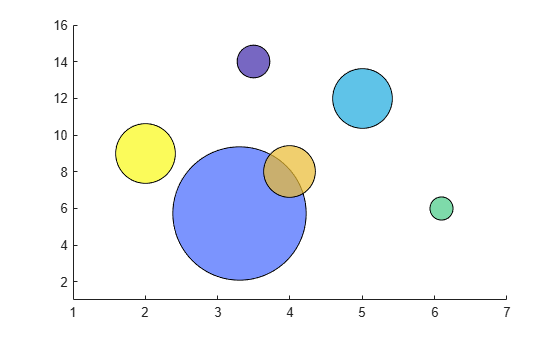lines

Lines colormap array

Description

c = lines returns the lines colormap as a three-column array with the same number of rows as the colormap for the current figure. If no figure exists, then the array contains 256 rows. Each row in the array contains the red, green, and blue intensities for a specific color. The intensities are in the range [0,1], and the color scheme matches the default ColorOrder property of the Axes. Thus, you can use this colormap to make your chart colors match the output of the plot function.example

c = lines(m) returns the colormap with m colors.

Examples

collapse all

Create a scatter plot using the default colors.

x = [3.5 3.3 5 6.1 4 2];
y = [14 5.7 12 6 8 9];
sz = 100*[6 100 20 3 15 20];
c = [1 2 3 4 5 6];
scatter(x,y,sz,c,'filled','MarkerEdgeColor','k','MarkerFaceAlpha',.7);
xlim([1 7]);
ylim([1 16]);Get the lines colormap array with six entries. Then replace the colormap in the scatter plot.

c = lines(6);
colormap(c);Input Arguments

collapse all

Number of colors, specified as a scalar integer value. The default value of m is equal to the length of the colormap for the current figure. If no figure exists, the default value is 256.

Data Types: single | double

Compatibility Considerations

expand all

Behavior changed in R2019b